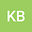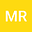Starlikness Associated with Euler Numbers
••• Mohsan RazaLet $\psi _{E}\left( z\right) =\sec h\left( z\right) =\underset{n=0}{\overset% {\infty }{\sum }}\frac{E_{n}}{n!}z^{n},$ where the constants $E_{n}$ are Euler numbers. The class $\mathcal{S}_{E}^{\ast }$ denote the class of normalized analytic functions $f$ satisfying $zf^{\prime }(z)/f(z)\prec \psi _{E}\left( z\right)$. For this class, we obtain structural formula, inclusion results, and some sharp radii problems such as radius of convexity, radius for the class of Janowski starlike functions and radius for some other subclasses of starlike functions. We also find sharp coefficient results and sharp Hankel determinants for functions in the class $\mathcal{S}_{E}^{\ast }.$